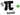## 激情碰撞中的圆周率

Wed, 23rd October 2019Edit on Githubbubbles

...

$\frac{1}{2}m_1v_1^2+\frac{1}{2}m_2v_2^2=\frac{1}{2}m_1v_1'^2+\frac{1}{2}m_2v_2'^2, m_1v_1 + m_2v_2 = m_1v_1' + m_2v_2'$

$v_1'=\frac{v_1(m_1-m_2)+2m_2v_2}{m_1+m_2},v_2'=\frac{v_2(m_2-m_1)+2m_1v_1}{m_1+m_2}$

$A=( \begin{array}{cc} \frac{m_1-m_2}{m_1+m_2} & \frac{2 m_2}{m_1+m_2} \\ \frac{2 m_1}{m_1+m_2} & \frac{m_2-m_1}{m_1+m_2} \\ \end{array} ), ( \begin{array}{c} v_1' \\ v_2' \\ \end{array} )=A ( \begin{array}{c} v_1 \\ v_2 \\ \end{array} )$

$B = \left( \begin{array}{cc} -1 & 0 \\ 0 & 1 \\ \end{array} \right)\cdot A= \left( \begin{array}{cc} \cos (2 \theta ) & -2 \cos ^2(\theta ) \\ 2 \sin ^2(\theta ) & \cos (2 \theta ) \\ \end{array} \right)$

n轮碰撞[方块之间的碰撞+墙壁反弹]之后,

$B^n = \left( \begin{array}{cc} \cos (2n\theta) & -\cot (\theta)\sin(2n\theta) \\ \tan(\theta) \sin(2n\theta) & \cos(2n\theta) \\ \end{array} \right)$

$( \begin{array}{c} v_1^{Final} \\ v_2^{Final} \\ \end{array} )=B^n ( \begin{array}{c} v_1^{initial} \\ v_2^{initial} \\ \end{array} )=B^n ( \begin{array}{c} 0 \\ -1 \\ \end{array} )$

Github